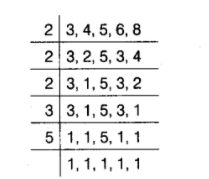# Find the least square,

Question:

Find the least square, number, which is exactly divisible by 3, 4, 5, 6 and 8.

Solution:

The least square number divisible by each of $3,4,5,6$ and 8 , is equal to the LCM of $3,4,5,6$ and 8 .$\therefore$ LCM of $3,4,5,6$ and $8=2 \times 2 \times 2 \times 3 \times 5=120$

The prime factorisation of $120=(2 \times 2) \times 2 \times 3 \times 5$

Here, prime factors 2,3 and 5 are unpaired. Clearly, to make it a perfect square, it must be multiplied by $2 \times 3 \times 5$, i.e. 30 .

Therefore, required number $=120 \times 30=3600$ Hence, the least square number is 3600 .